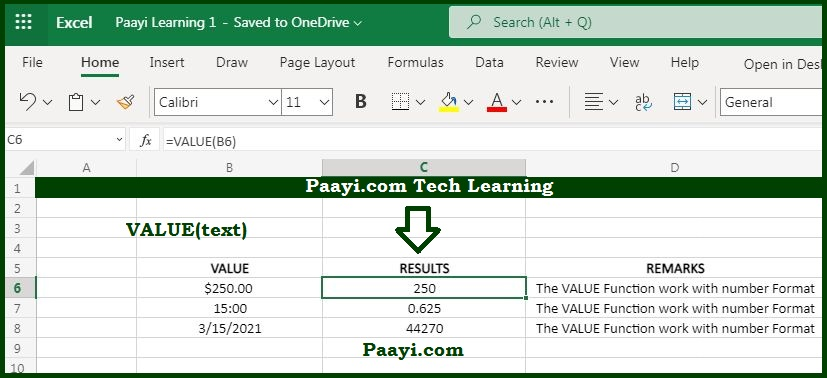# Learn How to use Microsoft Excel VALUE Function

Written by | 0 Comments | 828 Views

In this article, you will learn how to use the Microsoft Excel UPPER function and its prime function in Microsoft Excel. You will also get to know the Microsoft Excel UPPER function return value and syntax with the help of some examples.

### Microsoft Excel VALUE Function

The MS Excel VALUE function is used to convert text as a number. That means with the help of the VALUE function; you can able to convert text that appears in a recognized format as - date, number, or time format, into a numeric value. It should be noted here that the VALUE function is not required in Excel because Excel automatically converts text to a numeric value.

### VALUE Function Return Value

It will return a numeric value.

### VALUE Function Syntax

= VALUE(text)

Where the argument:

• text: It is the text value you want to convert into a number.

## How to Use the Microsoft Excel VALUE Function?As we know, the VALUE function converts text to a numeric value, and it will convert the text that appears in a recognized format. But Excel is also provided with the in-built function where the Excel automatically converts the text into a numeric value, but as per Microsoft, the VALUE function is provided for compatibility with the other spreadsheets programs.